# near point

closest point on optical axis with an image that has „acceptable sharpness“Where CoC is the Circle of Confusion (the largest accepted Airy-disk) in Millimeter.

Alternatively, we can express the NearPoint using the magnification M :If we use for example a 1/2.5″ 5 Aptina Megapixel greyscale Sensor mit 2.2pixel pitch, we can use the pixel diagonal as CoC for crisp images, sayA 5 Mega lens with f=7.2mm focal length and F-stop F2.4, focused to an object distance of 100mm then has a far point ofund einen Nahpunkt von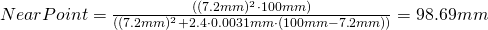and thusIf instead we use a 5 Megapixel greyscale Sony Sensor with 3.45pixel pitch, we can choose as CoC the diagonal of the pixel for crisp images, sayA 5 Mega lens with f=7.2mm focal length and F-stop F2.4, focussed to 100mm then results inmm
and a nearpoint ofthus we getIf we use a color sensor instead we can usefor crisp images. For the two sensors above we then get: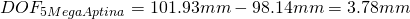To increase the DOF we can increase the Pixel Size, but we either lose resolution, or (at the same pixel count) the magnification changes)
If you change the focal length of a lens in a way, that (with the same sensor) you get the same FOV (then from a different distance) this results in the same DOF !!!
To increase the DOF we can keep the working distance and the pixel size while reducing the sensor size, but then we lose resolution (because less of the same size pixels fit on the now smaller sensor)
When a lens is focussed to the hyperfocal distance H, the far point is atand the near point is at.
The DOF isthen, thus focussing to the hyperfocal distance results in the largest possible DOF.

Yet another way to express the Near-Point is to use the Hyperfocal distance H :If the lens is then focused to some object distance OD, this OD can be expressed as fraction of H :or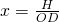Then the near point is,

the far point isand the Depth of field isWhen we set the object distance OD to the hyperfocal distance H, we get:Therefore fromwe get.
This means however, thatand therefore,For a lens with f=12mm, F#=2.8 and a CoC of 3.1um we get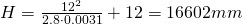Forwe getSo, withandwe get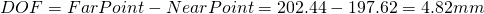Forwe getSo, withandwe getTherefore by decreasing the OD by factor 2, the DOF decreased by factor 4.

When the magnification increases by factor 2, the DOF decreases by factor 4

or, equivalent :

When the object distance decreases by factor 2, the DOF decreases by factor 4

# Newton’s Rings

Netwon’s Rings are a phenomenon of wave optics and occur if there is a small path difference of coherent light.
They occur for example for large spheres on a glass plate with a very small gap between sphere and plate.

Some light is reflected from the glass sphere’s surface and some is reflected from the glass plate’s surface.
Because the light is coherent, we get constructive and destructive interference.

For the gap being 1/4 Lambda, the path in the gap and back is lambda/2, which results in destructive interference. Same for path lengths in the gap of 3/2 lambda, 5/2, etc. Say, for gaps that are odd multiples of lambda 1/4.
For even multiples of lambda/4, say lambda/2 , 3/2 Lambda etc, we get constructive interference.

Say, we simply can count the bright rings. For 666nm red light for example, three rings are 1000nm = 1 micrometer, which is the length of the air gap.

Newton’s rings are used in lens production, together with interferometers to find how close the current shape is from the wanted shape.

Thus surface accuracies are given in “(Newton’s) Rings”. The less rings, the better the shape got to be (and the more expensive the lens will be). Also a diametre should be given, for example five rings in 18mm diameter.

According to ISO DIN 10110 the default specs are: Wavelength 546,07 nm, 5 Rings at at 10 mm clear aperture

Below is a version of Newton Rings occuring between a sphere under test and a reference sphere

# Newtonian Image EquationImage formation of a lens. The values z und z’ are marked red.

The Newtonian equation is an equation of ray optics named after the English physicist Isaac Newton.withandSolved for ObjectDistance we get:Solved for ImageDistance we get:This Newtonian Image equation is often used instead of the “lens equation”. Here z is the differences between object distance and focal length and z ‘ is the difference between image distance and focal length.

Be an object point 20mm left of the first Focal point of a lens of 30mm focal length.
Then it’s image isto the right of the second Focal point (“-” 20 because the left of the focal point).
Be an object point 10mm left of the first Focal point of a lens of 30mm focal length.
Then it’s image isto the right of the second focal point (“-” 10 because the left of the focal point).

The advantage of Newton’s equation is that you can determine the focal point and distances from these focal points of lenses relatively easy, while principal points can be relatively difficult to determine.